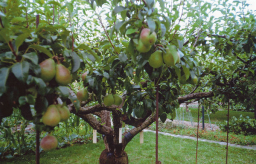# Distances 64024

Eleven trees are planted on the street at equal distances. If they planted three more trees, the spaces between them would be 12 m shorter. What is the distance in meters between the first and the last tree?

x =  520 m

### Step-by-step explanation:Did you find an error or inaccuracy? Feel free to write us. Thank you!

Tips for related online calculators
Do you have a system of equations and looking for calculator system of linear equations?
Do you want to convert length units?